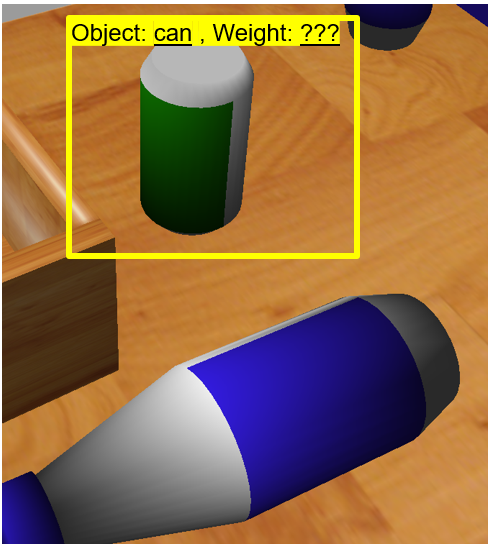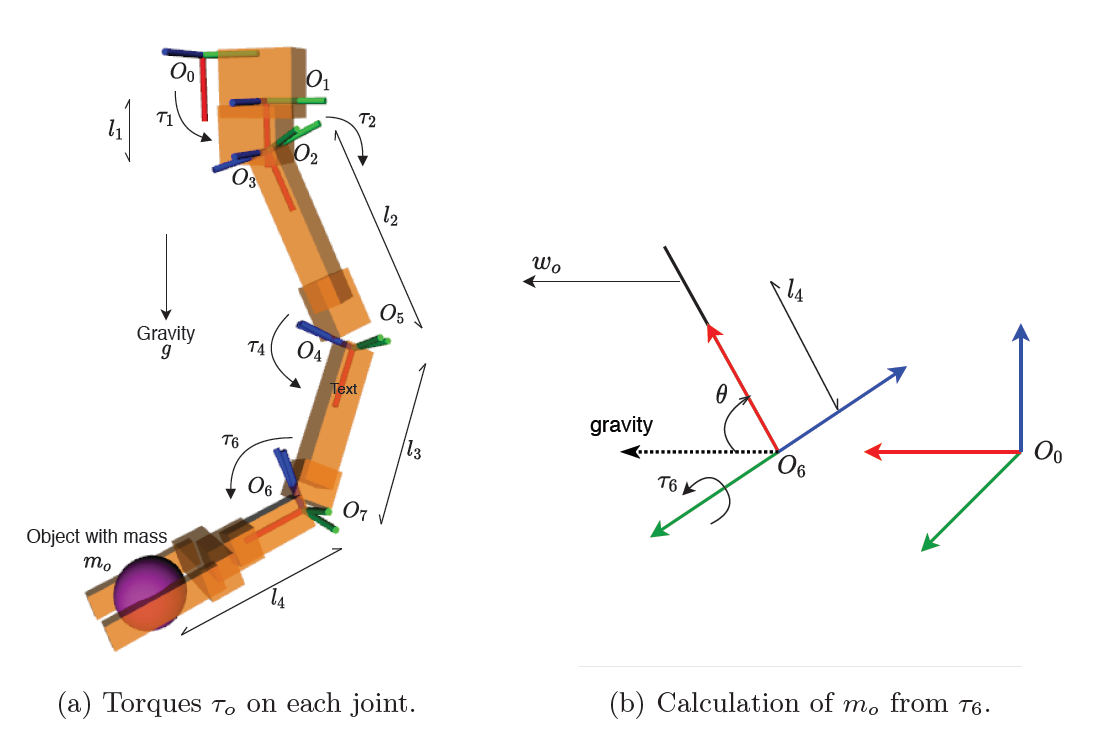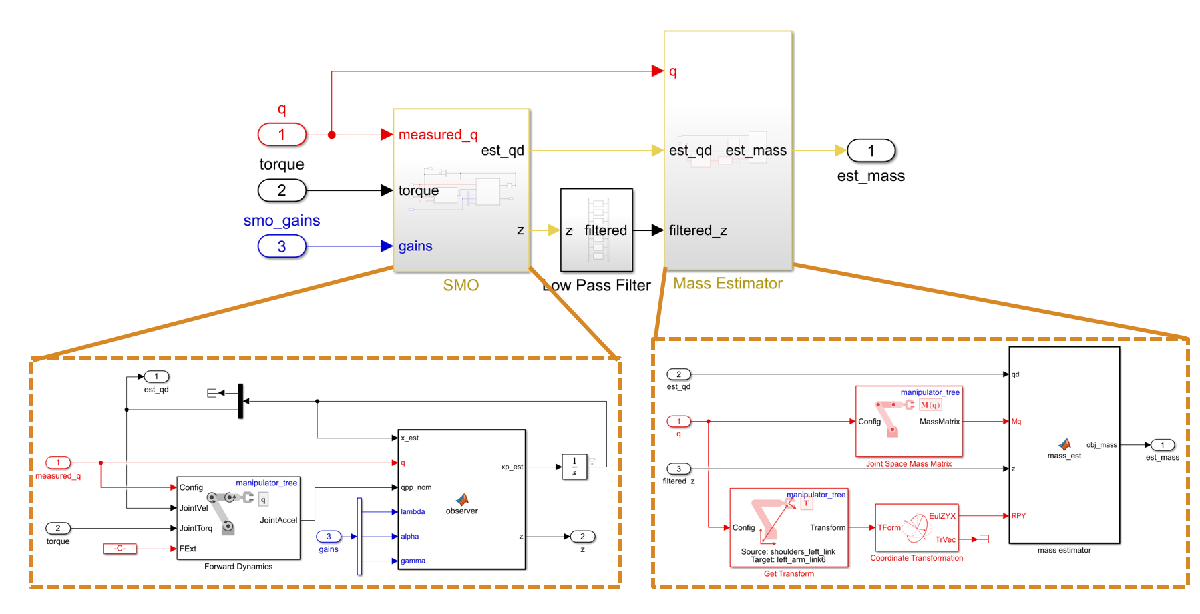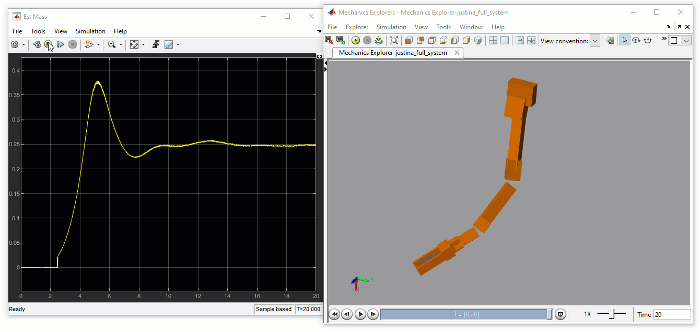# Weight Perception for Robot Manipulators

In today’s post, Jose Avendano joins us to talk about a perception algorithm developed specifically for robot manipulators to determine the weight of grasped objects. Over to you, Jose..
Robot hands, more formally referred to as robot manipulators, are an active field of research in the robotics community. In this blog, we will cover a perception algorithm developed specifically for robot manipulators to determine the weight of grasped objects. In general, more information about the environment a robot operates on, will lead to more versatile and robust algorithms. This research is currently published with accompanying files and was developed by the Bio-robotics Laboratory at the Universidad Nacional Autónoma de México. In this blog, we will provide a brief introduction to technical aspects of the research and how to use these algorithms in any robotics project.

## Inspiration (Service robots)

The inspiration for this weight estimation system comes from one of the leagues in the RoboCup competition. Domestic service robots that participate in this competition are tasked with finding household items and retrieving them from multiple locations. However, some of the objects such as cans can look the same but be different… They can be empty! This is something that a human can easily determine after picking them up, however robots need help learning how to identify these different states of common objects. Knowing the weight of an object will compliment a perception algorithm that might otherwise be only based on visual input. However, weight estimation research is not only applicable to service robotics. Similar algorithms are equally valuable for any autonomous system applications like human-machine interaction, manufacturing, construction, medical devices, and military applications.## New Insights from Existing Sensors

At this point you might ask yourself, if you really need to know the weight of something that was grasped by a robot, why not just add a load sensor to your robot? (Like the ones in your bathroom scale).
You could. But the whole point of this research is to demonstrate that you don’t need to add any more devices or complexity to your robot. Instead, you can use existing information from your robot joints, and some mathematics, to virtually get the same result. Simplifying it some more, if you know the weight of your robot components, you should be able to teach the robot how to quantify the excess weight it is carrying.
Here is what you would need to implement this virtual sensor:
• Robot parameters (Geometry, mass, and inertia of robot components)
• Joint Sensor Information (Torque and position of each robot joint)## Implementation

Here is where the explanation of the algorithm becomes more involved. To find the fault (error) in the system, which is proportional to the excess weight, we first must calculate the expected states of the system at a given configuration. Then based on the expected torque to hold the manipulator’s configuration, we can calculate the torque exerted by the object on the manipulator joints. For all of this to be robust, it is necessary to use an optimal state estimation method. In this implementation, a Sliding-Mode Observer is synthesized using the manipulator’s parameters to reconstruct the necessary disturbance term. If you are interested in how the math is derived, you can refer to the publication. In short, sliding-mode observers are estimators that require a system model to predict system outputs, then they compare such predictions with measurements to correct estimations. Normally these models would be derived in a state space representation, but to make the algorithm more reusable the files posted are developed using Rigid Body Tree features. MATLAB RBT objects encapsulate a robot’s parameters, enabling common kinematics and dynamics algorithms and utilities to be implemented. The complete system ends up being a combination of a sliding-mode observer, a low-pass filter, and transformation of the error detected using the robot geometry as seen on the Simulink model below.## Modeling and Simulation

The algorithm was first developed using simulations before testing on a real robot. For this, the robot structure was imported to Simscape Multibody to be able to model the physics and interactions between the robot components. The simulated plant allows for discretely assigning the extra weight of the grasped object by using a variable mass component. Using this simulation, the algorithm designer can evaluate performance parameters of the estimation like accuracy, settling time, and even test for robustness by assigning error sources like sensor quantization. For testing purposes, the simulation stack also has a robot joint controller. Depending on the use-case and robot that the algorithm is adapted to, the joint controller subsystem can be bypassed on simulation by using ideal actuators, or custom controllers from other sources such as dedicated ROS nodes can be integrated. One thing that has to be kept in mind when implementing this weight estimator, is that it relies on setting an accurate distance measurement from the grasping location to the first joint up the kinematic chain. This means that the weight estimation accuracy depends on the robot grasping the objects consistently within the designated distance from the joint.## Uses and Reusability

An integral part of this research collaboration was to provide a reusable package for the robotics community. The algorithm can be adjusted to work with any manipulator by importing a robot description to MATLAB. This can be done by importing an existing URDF file or directly creating a rigid body tree object. Then it is necessary to pick the nearest joint to the end effector whose rotation axis is perpendicular to the gravity vector. With that information and the updated rigid body tree, the algorithm can be tested for the new robot platform and ROS nodes can be generated for the desired subsystems in order to integrate the weight estimation into complex robotics systems. We hope you found this research recap useful, and if you are interested in learning more, demo files are available along with the publication.

|